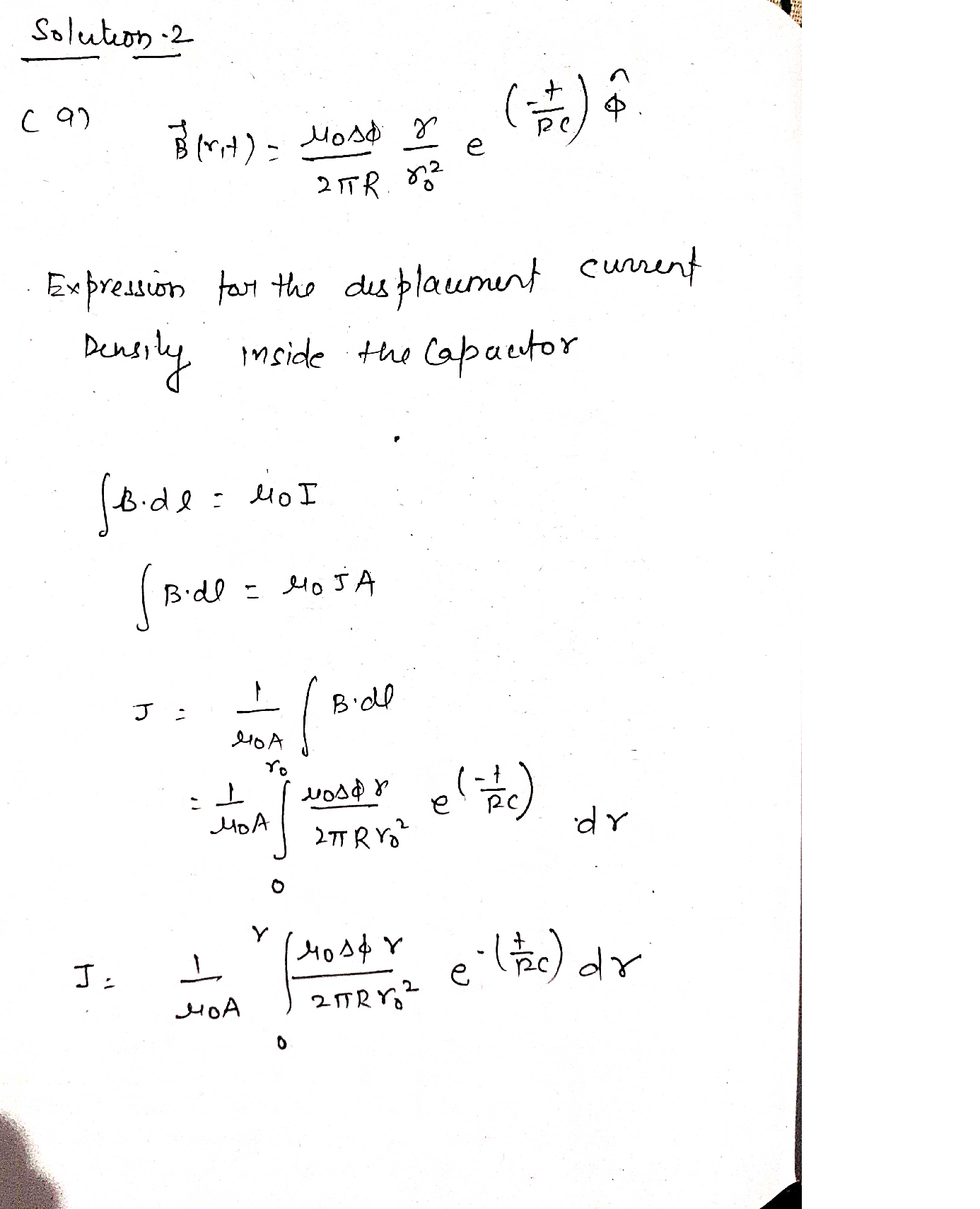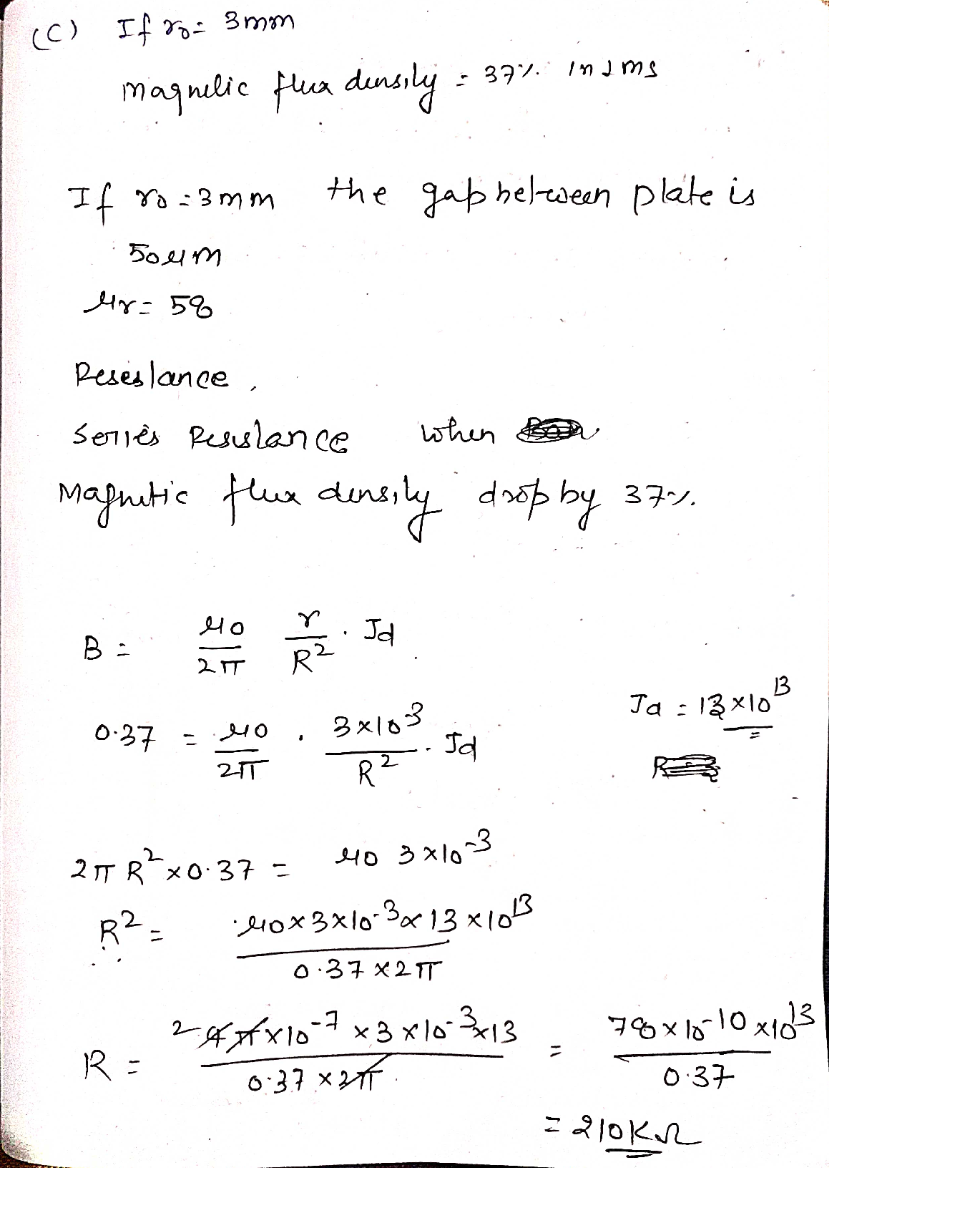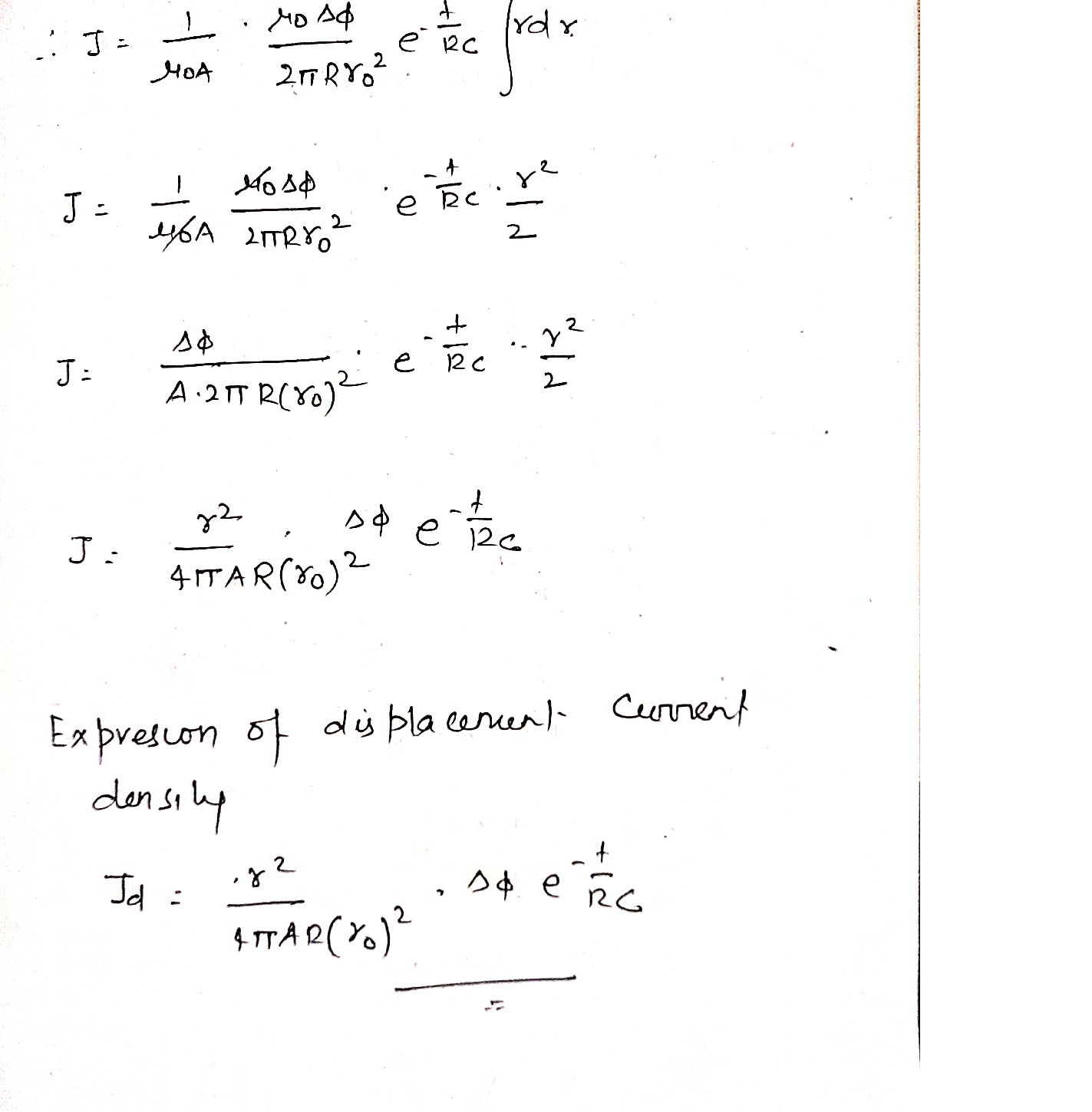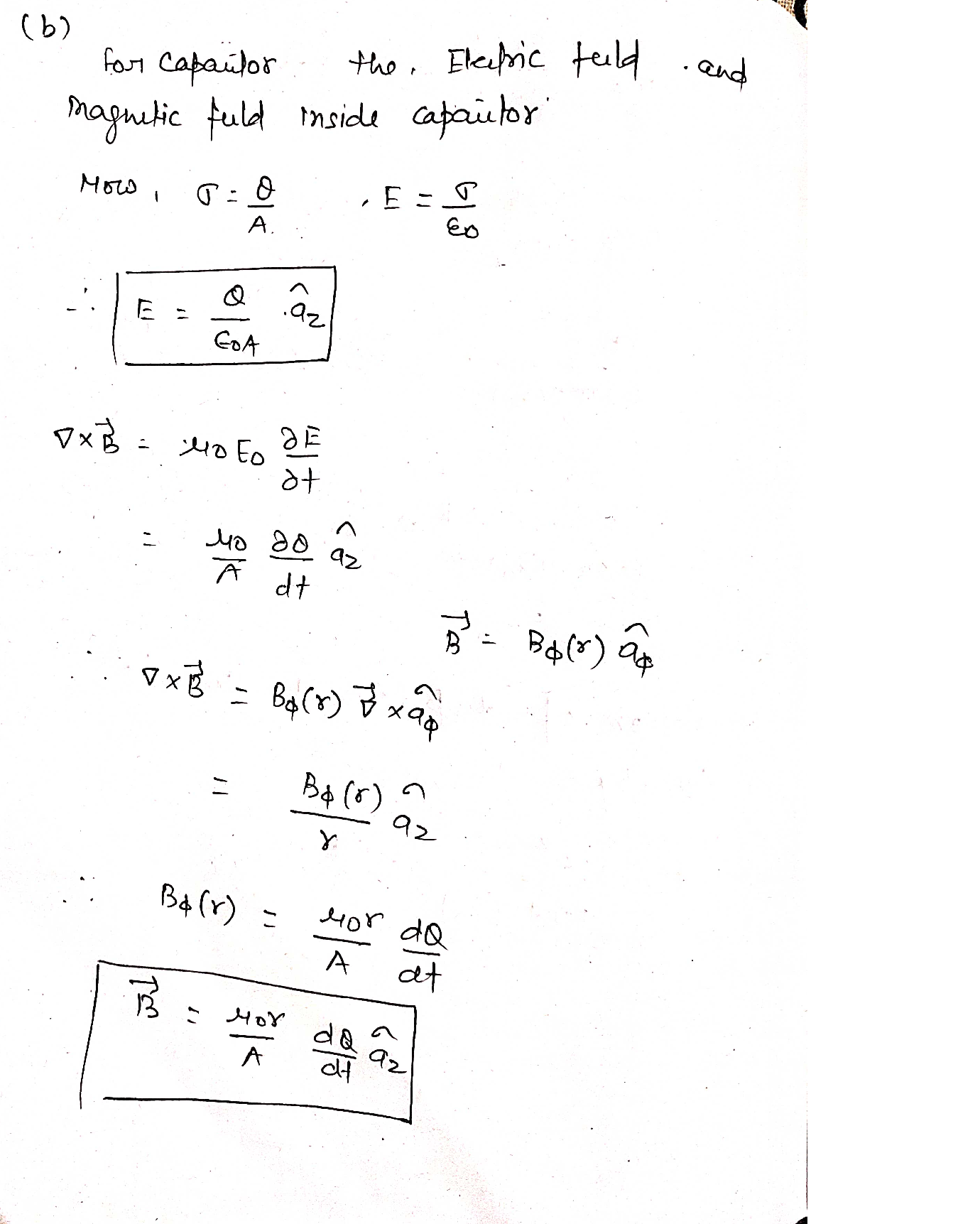Question

Mechanics

2) In charging a circular parallel plate capacitor, it can be shown that the magnetic flux density between the plates is of the form

\vec{B}(r, t)=\frac{\mu_{0} \Delta \phi}{2 \pi R} \frac{r}{r_{0}^{2}} \exp \left(-\frac{t}{R C}\right) \hat{\varphi}

where R is a resistance in series with the capacitor, C is the capacitance of the parallel plate capacitor of radius ro, r is the radial distance from the centre of the capacitor in cylindrical co-ordinates, t is time and A is the potential difference across the capacitor.

a) Using this result, determine an expression for the displacement current density inside the capacitor while it charges.

b) Consider the case where the material between the electrodes has a small but non-zero conductivity. Briefly,and with explicit reference to the appropriate Maxwell equations and their dependent variables such as current and time, describe the electric and magnetic fields present inside the capacitor, including any relevant information about the field directions.

c) It ro = 3 mm, the gap between the parallel plates is 50 µm and filled with a material with a relative permittivity 58,determine the series resistance required so that the magnetic flux density drops by 37% in 1 ms.Verified### Question 45701Mechanics

Q3 [45%] Consider the probability of finding a molecule in its ground state, P(e0),or first excited state, P(ɛ1), given the that energy separation between the two statesis 5 kJ/mol. You may assume that the states are non-degenerate (go = g1 = 1).
3] Calculate BAE for this system at 300 K.
[15%] Calculate the ratio of the excited and ground state populations P(ɛ1)/P(ɛo) at temperature T = 300 K
3. [15%] Show (by calculation) what would happen to the ratio if the temperature was increased by a factor of two.

### Question 45700Mechanics

Q2 [30%] Electron kinetic energies are often measured in units of electron-volts(1 eV = 1.6 x 10-19 J). When an aluminum plate is irradiated with UV light of 200nm wavelength, the ejected electrons are observed to have an average kinetic energy of about 2.11 eV. Use these results to
] Calculate the photon energy (in eV units).
Determine the electron binding energy O of aluminum (in eV units).

### Question 45699Mechanics

Q1 [25%] Consider a ball with mass m=5.0 kg on a hill with height h=10 m whichis inclined at an angle 0=30 degrees with respect to the horizontal axis and recallthat g=9.8 m/s².
] Calculate potential energy due to gravity11 1
Calculate the force on the ball due to gravity
Calculate the component of the force along the slope (z-axis).

### Question 45661Mechanics

What is the maximum mass of this water strider so that it can keep from breaking through the water surface? The strider has six legs

### Question 45660Mechanics

What is the maximum possible upward force on the foot due to surface tension of the water?

### Question 45659Mechanics

Blood plasma (at 37.0°C) is to be supplied to a patient at the rate of 2.80 × 10-6 m³/s. If the tube connecting the plasma to the patient's vein has a radius of 2.00 mm and a length of 60.8 cm, what's the pressure difference between the plasma and the patient's vein? Viscosity1.30 x 103 Pa·s.blood plasma is
39.55 Pa

### Question 45658Mechanics

Four identical sections of pipe are connected in various ways to pumps that supply water at the pressures indicated in the figure. The water exits at the right at 1.00 atm. Assume viscous flow. If the total volume flow rate in system Bis 0.0128 m³/s, what is the total volume flow rate in systemC?

### Question 45657Mechanics

Estimate the air speed above the wings. Density of air is 1.29 kg/m3.

### Question 45656Mechanics

An airplane flies on a level path. There is a pressure difference of 524 Pa between the lower and uppersurfaces of the wings. The area of each wing surface is about 100 m². The air moves below the wings ata speed of 80.5 m/s.
Estimate the weight of the plane.

### Question 45655Mechanics

The average speed of blood in the aorta is 0.320 m/s, and the radius of the aorta is 1.00 cm. There are about 2.00 × 10° capillaries with an average radius of 6.49 µm. What is the approximate average speed of the blood flow in the capillaries?

### Submit query